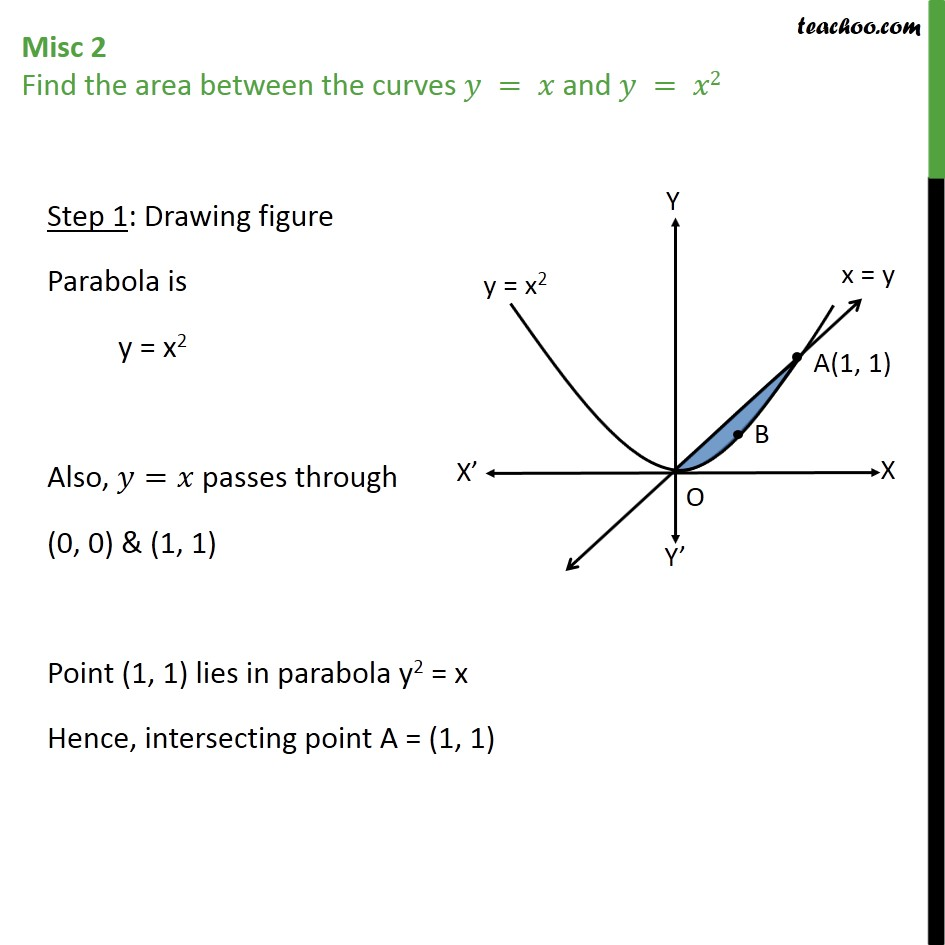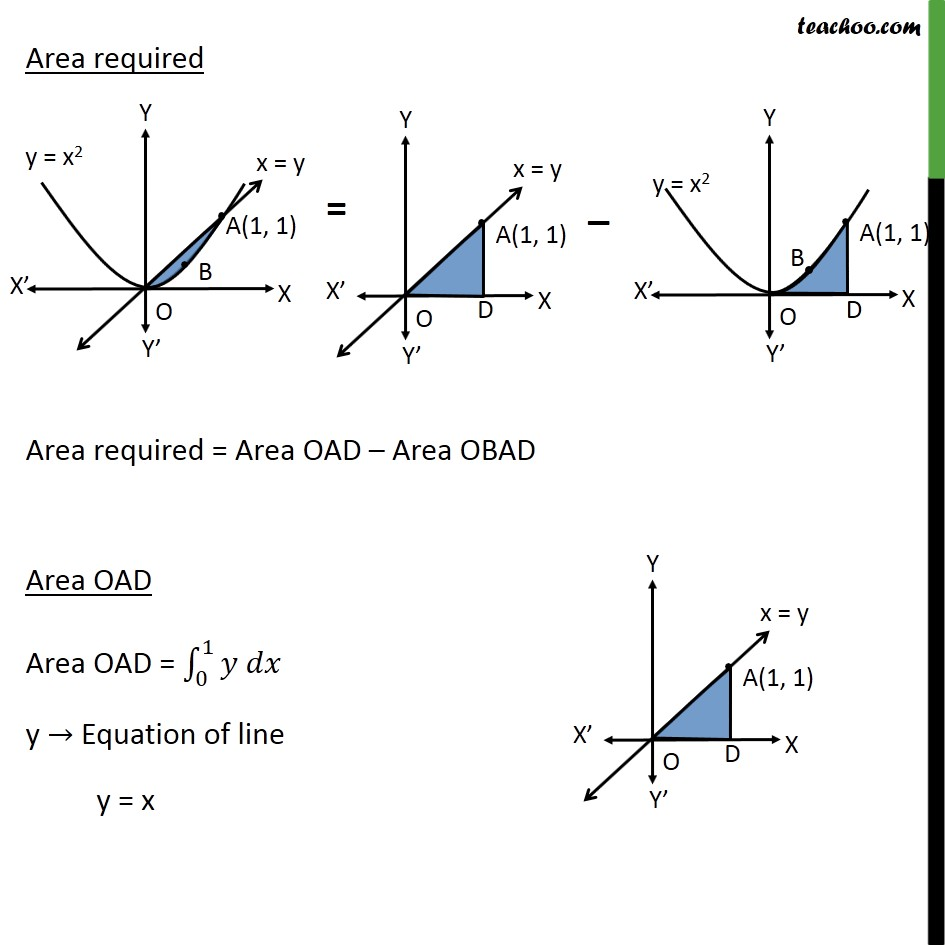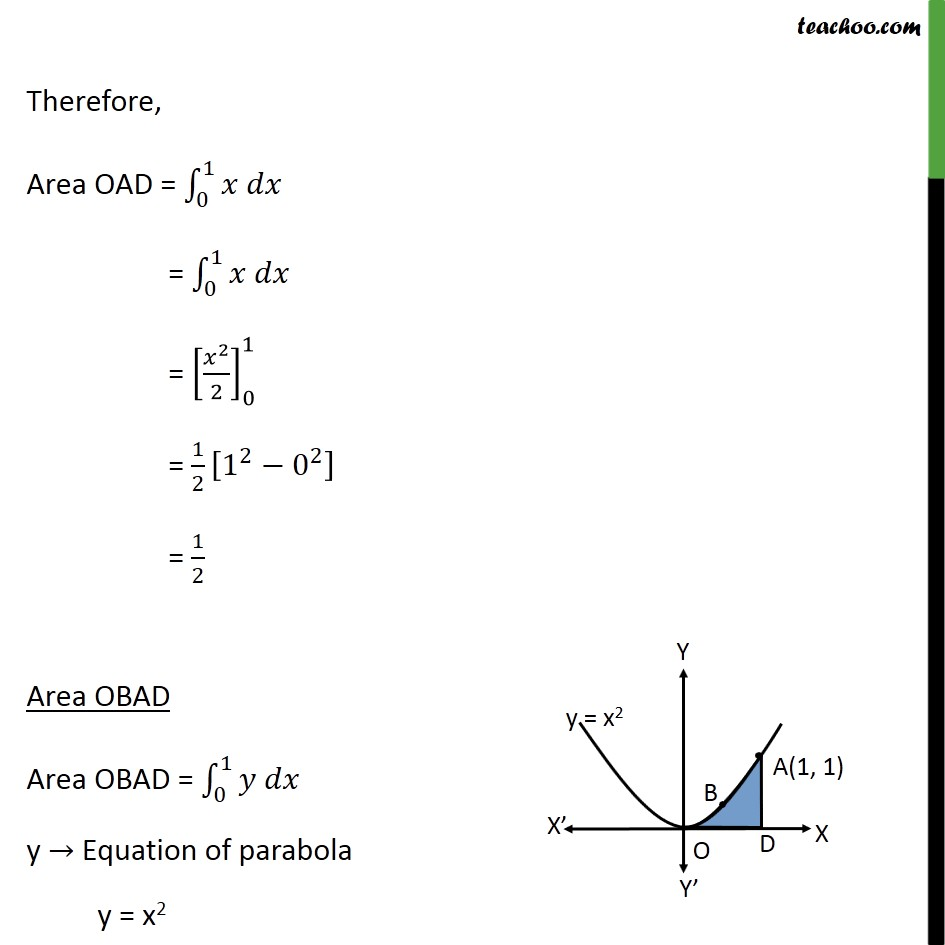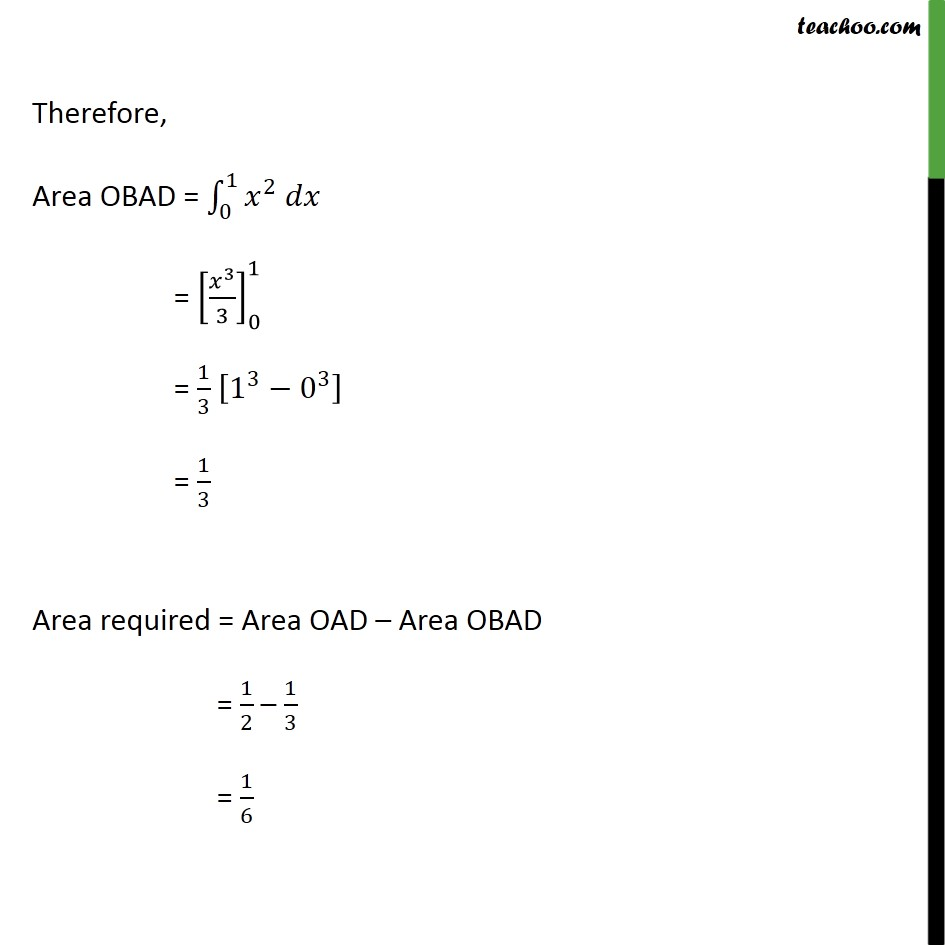1. Chapter 8 Class 12 Application of Integrals
2. Serial order wise
3. Miscellaneous

Transcript

Misc 2 Find the area between the curves 𝑦 = 𝑥 and 𝑦 = 𝑥2 Step 1: Drawing figure Parabola is y = x2 Also, 𝑦=𝑥 passes through (0, 0) & (1, 1) Point (1, 1) lies in parabola y2 = x Hence, intersecting point A = (1, 1) Area required Area required = Area OAD – Area OBAD Area OAD Area OAD = 0﷮1﷮𝑦 𝑑𝑥﷯ y → Equation of line y = x Therefore, Area OAD = 0﷮1﷮𝑥 𝑑𝑥﷯ = 0﷮1﷮𝑥 𝑑𝑥﷯ = 𝑥﷮2﷯﷮2﷯﷯﷮0﷮1﷯ = 1﷮2﷯ 1﷮2﷯− 0﷮2﷯﷯ = 1﷮2﷯ Area OBAD Area OBAD = 0﷮1﷮𝑦 𝑑𝑥﷯ y → Equation of parabola y = x2 Therefore, Area OBAD = 0﷮1﷮ 𝑥﷮2﷯ 𝑑𝑥﷯ = 𝑥﷮3﷯﷮3﷯﷯﷮0﷮1﷯ = 1﷮3﷯ 1﷮3﷯− 0﷮3﷯﷯ = 1﷮3﷯ Area required = Area OAD – Area OBAD = 1﷮2﷯ – 1﷮3﷯ = 1﷮6﷯

Miscellaneous

About the AuthorDavneet Singh
Davneet Singh is a graduate from Indian Institute of Technology, Kanpur. He has been teaching from the past 9 years. He provides courses for Maths and Science at Teachoo.